A review of the timing of the most publicized AI advances suggests that perhaps many major AI breakthroughs have actually been constrained by the availability of high-quality training data sets, and not by algorithmic advances.

Moreover, the preference of high-quality training data sets over purely algorithmic advances might allow an order-of-magnitude speedup in AI breakthroughs.

However, getting this data is neither an easy nor a cheap task, Mechanical Turk tagging data-sets campaigns could cost hundreds of dollars easily and yet with an uncertain quality. Therefore, the question is how to exploit the minimal data we have and still be able to learn well (generalize)

Let’s take a simple real captcha (one of the old generation but some sites actually use them still) and try to break it.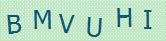The naive way to solve this problem would be to take a big data-set of captchas, similar to as above, label them manually, and implement a convolutional neural network for image classification. That is doable of-course but you need tons of labeled data for achieving a good accuracy.

A simple problem requires a simple approach right? Let’s try a different simple approach (:
##### Generating letter images using Linux fonts:
• We will use the English alphabet letters (but this can be applied to any language)
``letters = list('abcdefghijklmnopqrstuvwxyz')``
• Generating the images:
``````letters_folder = "letters"
fonts = []
for dirpath, dirnames, filenames in os.walk("/usr/share/fonts/truetype"):
for filename in [f for f in filenames if f.endswith(".ttf")]:
fontPath = os.path.join(dirpath, filename)
fonts.append(fontPath)

if not os.path.exists(letters_folder):
os.makedirs(letters_folder)

for fontPath in fonts:
fontName = fontPath.split('/')[-1][:-4]
font = ImageFont.truetype(fontPath, 20)
for l in letters:
img = Image.new("RGBA", (32,32),"black")
draw = ImageDraw.Draw(img)
draw.text((5,5),l.upper(),"white",font=font)
img.save(letters_folder+"/"+l+"_"+fontName+".png")
```
```
Resulting in images such as these: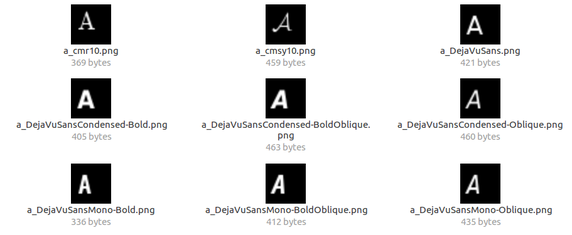This approach is simple yet powerful!
We just created a data set of letters based on the fonts in our machine. It allows us to have letters in all kind of shapes which helps to generalize the learning process.
Now lets add some noise to the images:
``````for i in range(1,100):	# Draw random points
draw.point((randint(0,32),randint(0,32)), fill=255)

# Blur the image
``````
Resulting in the following images: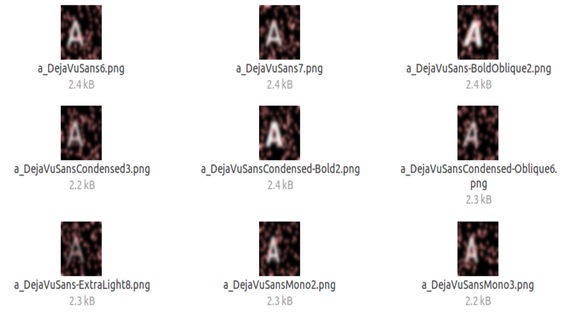#### Let’s create a model!

We want to create a model that would distinguish between the letters, so it’s a multi-class classification problem. We will be using Keras deep learning library . A CNN model could be applied here:
• The input is a (32,32) gray scaled image.
• The final layer is a soft-max function with 26 classes (26 english letters)
``````img_rows = 32
img_cols = 32
nb_classes = len(letters) # 26 letters

model = Sequential()

activation='softmax'))

model.compile(loss='categorical_crossentropy',
``````

#### Real-time data augmentation

Recently Keras released the ImageDataGenerator class that defines the configuration for image data preparation and augmentation.
This includes capabilities such as:
• Sample-wise standardization.
• Feature-wise standardization.
• ZCA whitening.
• Random rotation, shifts, shear and flips.
• Dimension reordering.
• Save augmented images to disk.
We will use ImageDataGenerator to augment the letters data set and then fine-tune the model with additional training on the combined data. ImageDataGenerator creates the images on the fly allowing to fit the model on batches with real-time data augmentation.
The goal by the augmentation process is to create “realistic” data
Using Keras ImageDataGeneretor generated the augmentated data:
``````# Augmentation - rotation & axis shifting
datagen = ImageDataGenerator(
rotation_range=10,
width_shift_range=0.5,
height_shift_range=0.2)
``````

#### Let’s train!

Training for 30 epochs on our generated letter images to measure accuracy gave the following plot, seemingly converging without much over-fitting: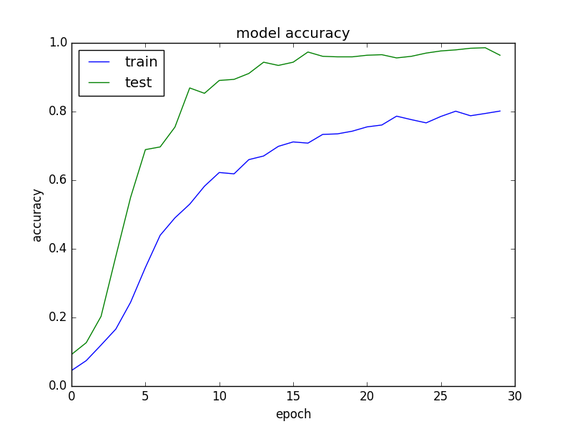Now that we got a trained model that can predict with good accuracy the right letters we can get back to our use case.

#### Back to our use case: breaking captchasOur captchas consist of 6 letters, so in order to detect the letters we will have to split the image into 6 parts where each part contains a letter:
``````numOfLetters = 6

im = 255-img

imgs = np.zeros((numOfLetters, 1, 32, 32), dtype=np.uint8)
t = np.floor(im.shape / float(numOfLetters))
bb = np.zeros((im.shape, 0), dtype=np.uint8) + 255

im1 = im.transpose()[0:int(np.floor(t))].transpose()
imgs[0, 0] = cv2.resize(np.concatenate((im1, bb), axis=1), (32, 32))
for i in range(1,numOfLetters-1):
from_pix = int(np.floor(i*t))
to_pix = int(np.floor((i+1)*t))
imi = im.transpose()[from_pix:to_pix].transpose()
imgs[i, 0] = cv2.resize(imi, (32, 32))

im_end = im.transpose()[int(np.floor((numOfLetters-1) * t)):].transpose()
imgs[numOfLetters-1, 0] = cv2.resize(np.concatenate((im_end, bb), axis=1), (32, 32))

fig, ax = plt.subplots(1,numOfLetters,figsize=(10,10))
for i in range(0,numOfLetters):
ax[i].imshow(imgs[i,0])
plt.show()

imgs = imgs.astype('float32') / 255.0
classes = model.predict_classes(imgs, verbose=0)
result = []
for c in classes:
result.append(self.letters[c])

prediction = ''.join(result).upper()

print("Prediction: "+ prediction)
``````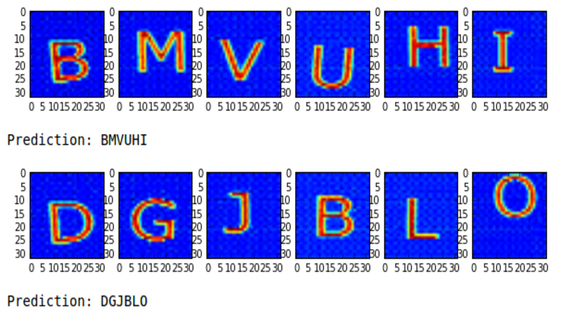Looks promising!

Out of 10 labeled captcha’s we got 5 correct, that’s pretty good but let’s improve that. Splitting the captcha into 6 parts worked well on some captchas and not so well on others:
The above image shows us that the model mistakenly thought that W was M. It seems that it has also mistaken between the pairs (C,G) and (B,P). All of those pairs indeed look similar, so in order solve this we will just make more of those letters!
As we said before ImageDataGenerator allows real time augmentation, therefore it is possible to change the parameters of the generator after we train. So in order to improve the model we changed the parameters via grid search method and trained again:
``````datagen = ImageDataGenerator(
rotation_range=20,
width_shift_range=0.5,
height_shift_range=0.7)

``````
Now we got 90% accuracy!

Note: solving this task didn’t require any data labeling procedure. Applying the “letters trick” along with the data augmentation process has been sufficient. How easy is that? (: